# Call DRC function in Ruby

Hi Sir,
I want to create a function in tool bar , and reference to other sample , I make that as below.
But , look like I can't call DRC functin in Ruby , May I know how to do that?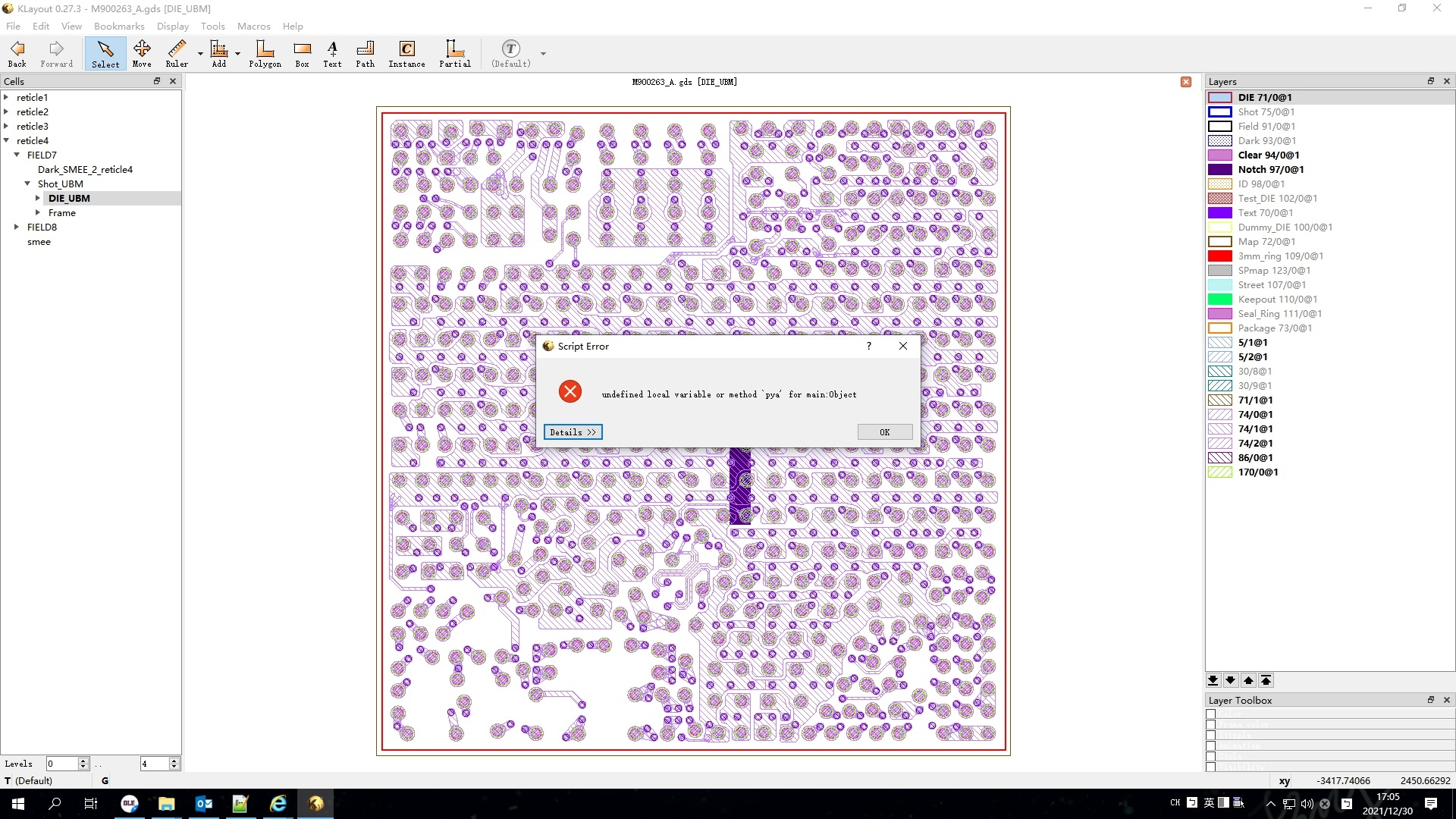``````class MenuAction < RBA::Action
def initialize( title, shortcut, &action )
self.title = title
self.shortcut = shortcut
@action = action
end
def triggered
@action.call( self )
end
private
@action
end

\$Check_bump_pitch = MenuAction.new( "Check min. bump pitch", "" ) do

app = RBA::Application.instance
mw = app.main_window

lv = mw.current_view
if lv == nil
raise "No view selected"
end

ly = pya.CellView.active().layout()

# equivalent of "a = input(7, 0)"
layer_a = ly.layer(94, 0)
UBM = pya.Region(ly.top_cell().begin_shapes_rec(layer_a))

layer_b = ly.layer(71, 0)
DIE = pya.Region(ly.top_cell().begin_shapes_rec(layer_b))

############################################

ubmcount=0
UBM.each {
ubmcount +=1
}

############################################
minPitch=Array.new
x=0
LayerList=[[0.0,0.0,0.25,0.25],[0.0,0.25,0.25,0.5],[0.0,0.5,0.25,0.75],[0.0,0.75,0.25,1.0] \
,[0.25,0.0,0.5,0.25],[0.25,0.25,0.5,0.5],[0.25,0.5,0.5,0.75],[0.25,0.75,0.5,1.0] \
,[0.5,0.0,0.75,0.25],[0.5,0.25,0.75,0.5],[0.5,0.5,0.75,0.75],[0.5,0.75,0.75,1.0] \
,[0.75,0.0,1.0,0.25],[0.75,0.25,1.0,0.5],[0.75,0.5,1.0,0.75],[0.75,0.75,1.0,1.0]]
LayerList.each { |n|
x1 = n.to_f
y1 = n.to_f
x2 = n.to_f
y2 = n.to_f

DIE.extent_refs(x1,y1,x2,y2).output(1000,x)
outline1=input(1000,x)
UBM.interacting(outline1).output(50000,x)
ubm1=input(50000,x)

outputdataX=Array.new
outputdataY=Array.new
pitchValue=Array.new

count=0
ubm1.each do |obj|
outputdataX << "#{obj.bbox.center.x.round(3)},#{obj.bbox.center.y.round(3)}"
outputdataY << "#{obj.bbox.center.x.round(3)},#{obj.bbox.center.y.round(3)}"
end

outputdataX.each do |out1|
point1 = out1.split(",")
xvalue1 = point1 
yvalue1 = point1 

outputdataY.each do |out2|
point2 = out2.split(",")
xvalue2 = point2 
yvalue2 = point2 
distance=Math.sqrt(( ( xvalue1.to_f - xvalue2.to_f ) *  ( xvalue1.to_f - xvalue2.to_f ))  +  (( yvalue1.to_f - yvalue2.to_f ) *  ( yvalue1.to_f - yvalue2.to_f ) ))
if distance > 0 then
pitchValue << distance.round(3)
end
end
end

minBumppitch = pitchValue.uniq.sort
minPitch << minBumppitch
#puts "Area #{x} ,min bump pitch is #{minBumppitch} ; layer is 50000/#{x}"
x+=1
}

RBA::MessageBox.info("min. Bump pitch", "The min. bump pitch is {minPitch.sort}", RBA::MessageBox.b_ok)
end

app = RBA::Application.instance
mw = app.main_window

``````

• You have to use `RBA::Region` instead of `pya.Region`. "pya" is Python, "RBA" is Ruby.

Plus, creating the Region in Ruby is done by using "new":

``````UBM = pya.Region(ly.top_cell().begin_shapes_rec(layer_a))
``````

I'd also recommend not to use upper-case variable names such as "UBM", because Ruby will treat these variables as constants.

Regards,

Matthias

• Hi Matthias,
After change the code as ..

``````ly = RBA::CellView.active().layout()
# equivalent of "a = input(7, 0)"
layer_a = ly.layer(94, 0)
ubm = RBA::Region(ly.top_cell().begin_shapes_rec(layer_a))

layer_b = ly.layer(71, 0)
die = RBA::Region(ly.top_cell().begin_shapes_rec(layer_b))
``````

I get error message as this one.
how can I do to debug this issue?
and , If it is a multi-top cell case , and I just want to process current cell view in script.
how to do that?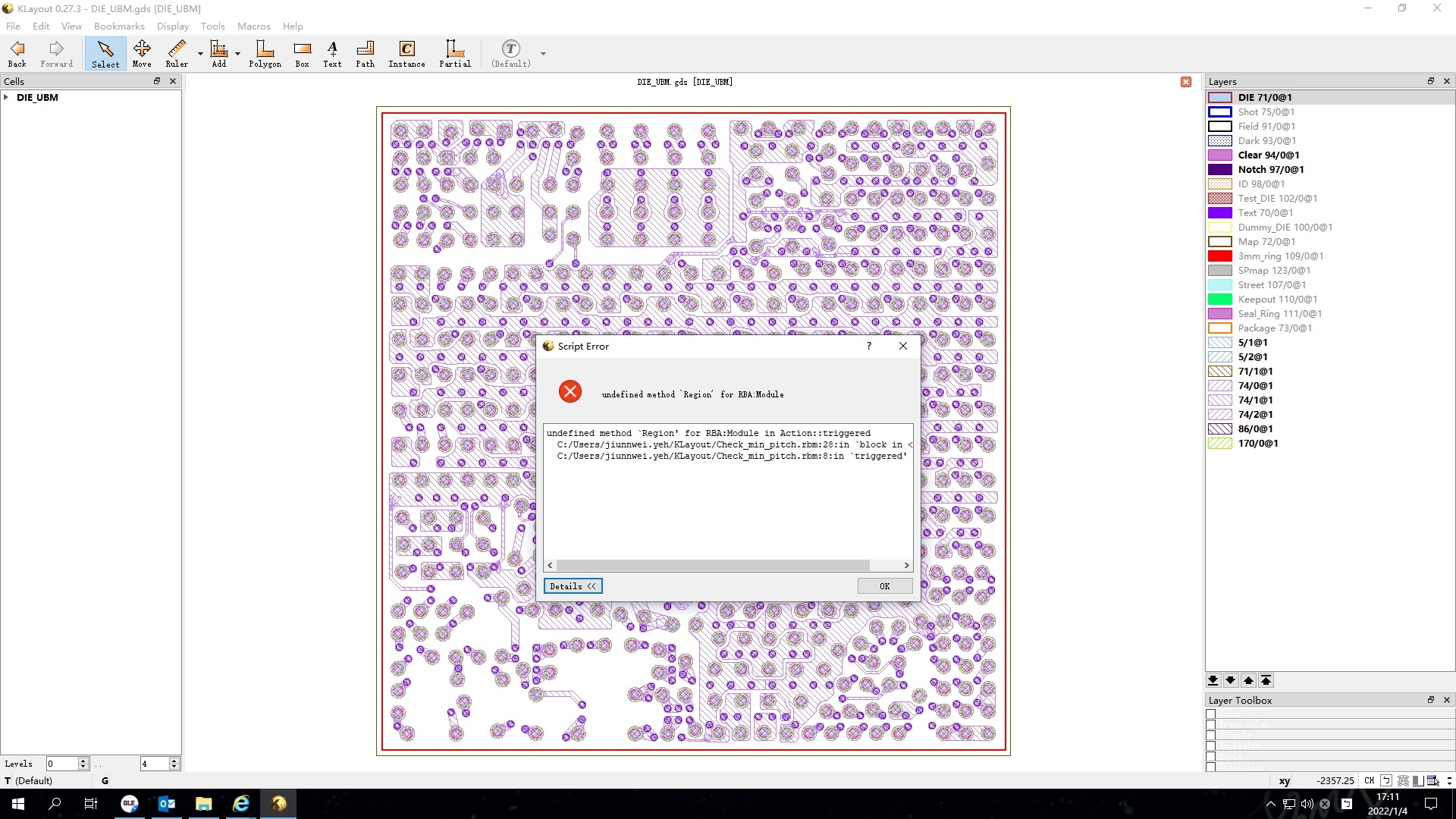• In Ruby, you need to call "new" to create a new object. So it's

``````ubm = RBA::Region::new(ly.top_cell().begin_shapes_rec(layer_a))
``````

in both places.

Matthias

• Hi Matthias,
after change code as..

``````ly = RBA::CellView.active().layout()
currentcellview = RBA::CellView.active().cell()
# equivalent of "a = input(7, 0)"
layer_a = ly.layer(94, 0)
ubm = RBA::Region::new(currentcellview.begin_shapes_rec(layer_a))
layer_b = ly.layer(71, 0)
die = RBA::Region::new(currentcellview.begin_shapes_rec(layer_b))
``````

I still get a message as attahced.
Thanks.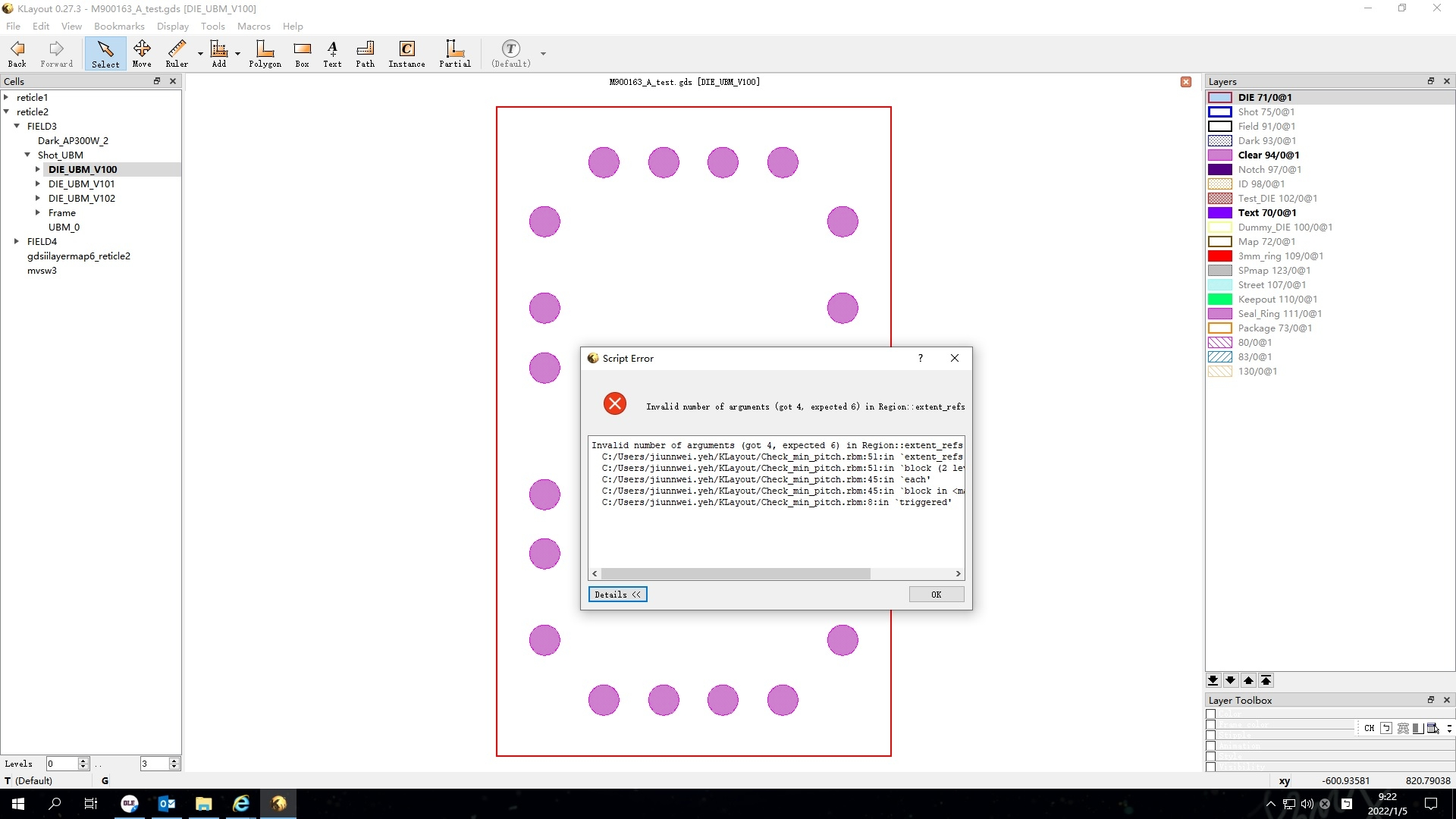• `Region#extent_refs` is an undocumented method for use in DRC's "extent_refs" implementation. It expects the four relative dimension arguments plus an x and y oversize value. You can set these values to 0 in your case:

``````DIE.extent_refs(x1,y1,x2,y2,0,0).output(1000,x)
``````

Matthias

• @Matthias
after change the code , it still get same result...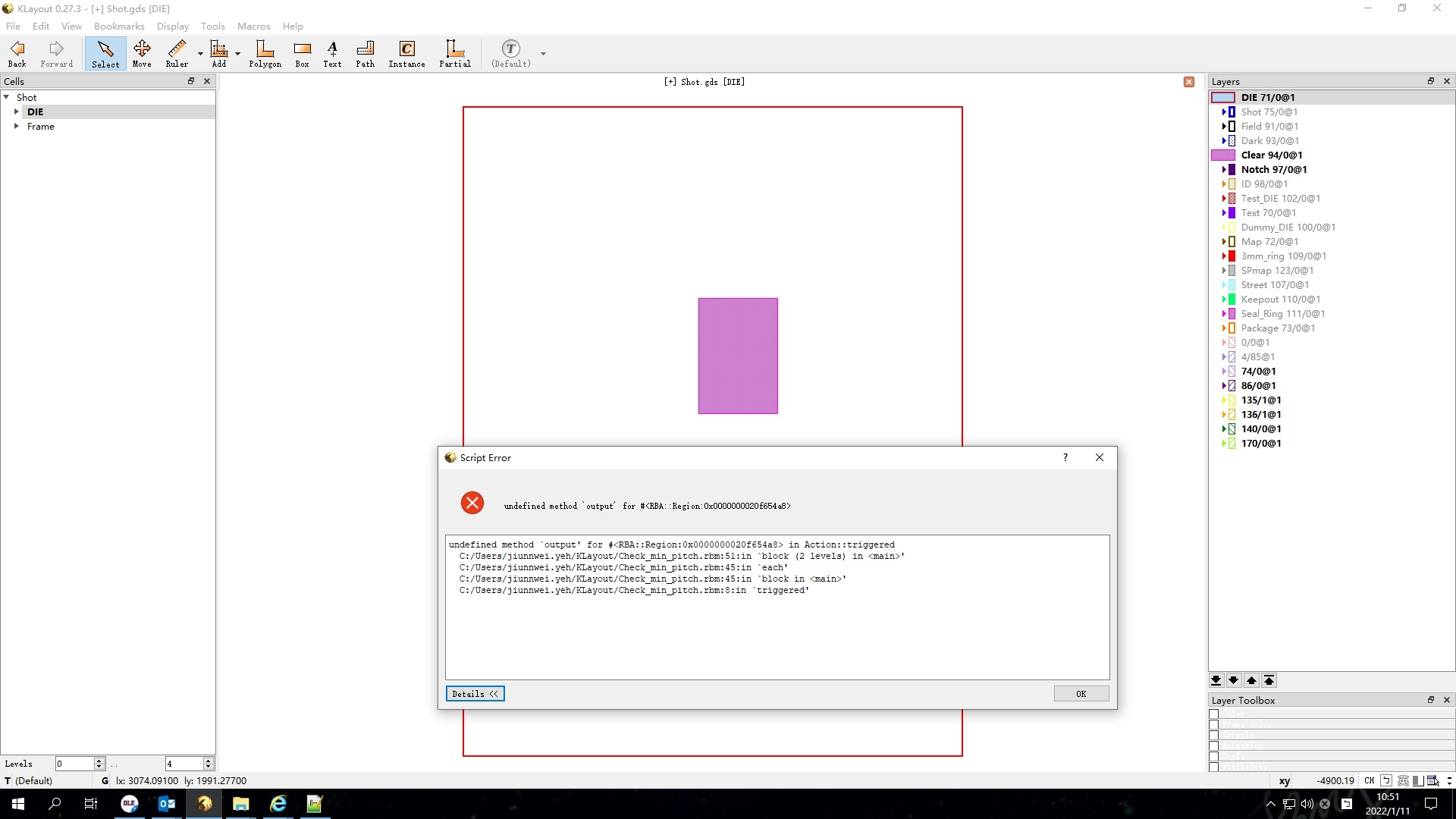``````class MenuAction < RBA::Action
def initialize( title, shortcut, &action )
self.title = title
self.shortcut = shortcut
@action = action
end
def triggered
@action.call( self )
end
private
@action
end

\$Check_bump_pitch = MenuAction.new( "Check min. bump pitch", "" ) do

app = RBA::Application.instance
mw = app.main_window

lv = mw.current_view
if lv == nil
raise "No view selected"
end
ly = RBA::CellView.active().layout()
currentcellview = RBA::CellView.active().cell()
# equivalent of "a = input(7, 0)"
layer_a = ly.layer(94, 0)
ubm = RBA::Region::new(currentcellview.begin_shapes_rec(layer_a))
layer_b = ly.layer(71, 0)
die = RBA::Region::new(currentcellview.begin_shapes_rec(layer_b))

############################################

ubmcount=0
ubm.each {
ubmcount +=1
}

############################################
minPitch=Array.new
x=0
LayerList=[[0.0,0.0,0.25,0.25],[0.0,0.25,0.25,0.5],[0.0,0.5,0.25,0.75],[0.0,0.75,0.25,1.0] \
,[0.25,0.0,0.5,0.25],[0.25,0.25,0.5,0.5],[0.25,0.5,0.5,0.75],[0.25,0.75,0.5,1.0] \
,[0.5,0.0,0.75,0.25],[0.5,0.25,0.75,0.5],[0.5,0.5,0.75,0.75],[0.5,0.75,0.75,1.0] \
,[0.75,0.0,1.0,0.25],[0.75,0.25,1.0,0.5],[0.75,0.5,1.0,0.75],[0.75,0.75,1.0,1.0]]
LayerList.each { |n|
x1 = n.to_f
y1 = n.to_f
x2 = n.to_f
y2 = n.to_f

die.extent_refs(x1,y1,x2,y2,0,0).output(1000,x)
outline1=input(1000,x)
ubm.interacting(outline1).output(50000,x)
ubm1=input(50000,x)

outputdataX=Array.new
outputdataY=Array.new
pitchValue=Array.new

count=0
ubm1.each do |obj|
outputdataX << "#{obj.bbox.center.x.round(3)},#{obj.bbox.center.y.round(3)}"
outputdataY << "#{obj.bbox.center.x.round(3)},#{obj.bbox.center.y.round(3)}"
end

outputdataX.each do |out1|
point1 = out1.split(",")
xvalue1 = point1 
yvalue1 = point1 

outputdataY.each do |out2|
point2 = out2.split(",")
xvalue2 = point2 
yvalue2 = point2 
distance=Math.sqrt(( ( xvalue1.to_f - xvalue2.to_f ) *  ( xvalue1.to_f - xvalue2.to_f ))  +  (( yvalue1.to_f - yvalue2.to_f ) *  ( yvalue1.to_f - yvalue2.to_f ) ))
if distance > 0 then
pitchValue << distance.round(3)
end
end
end

minBumppitch = pitchValue.uniq.sort
minPitch << minBumppitch
x+=1
}

RBA::MessageBox.info("min. Bump pitch", "The min. bump pitch is {minPitch.sort}", RBA::MessageBox.b_ok)
end

app = RBA::Application.instance
mw = app.main_window

``````
• It does not work this way ... "output" isn't a method of the Region class. It's a DRC method. The (flat) equivalent is to insert the Region into a Shapes object like this:

``````output_cell.shapes(output_layer_index).insert(region_to_output)
``````

Matthias

• @mathine
Do you have idea for how to make a cellS inputer?
As this code , I can enter 1 cell in input only. how to make multi input ?(I have many cells need to process...)

``````LayoutView = RBA::Application::instance.main_window.current_view.active_cellview.layout
dialog = RBA::QDialog.new( RBA::Application.instance.main_window)
dialog.windowTitle = "Creater"
layout = RBA::QVBoxLayout::new(dialog)
dialog.setLayout(layout)
eachcells = LayoutView.each_cell
die_list =Array.new()

eachcells.each do |ly|
die_list << ly.name
end

label =  RBA::QLabel.new(dialog)
label.text = "Choose DIE cell"
dieCell =  RBA::QComboBox.new(dialog)
die_list.each do |subcell|
end
dialog.exec
``````
• @jiunnweiyeh A QComboBox cannot be used to select multiple cells. You'll need a QListWidget for this (https://doc.qt.io/qt-5/qlistwidget.html).

Matthias

• @Matthias
Got it , Thanks your help ,
as this code , I can choose 1 cell in list only , how can I choose multi cell in the list?
(even I clicked the cell item with "Shift" key , I still can't select multi -cell in the list....)
And , how to get the values (cell name list as this case) after we choose that?

``````layoutView = RBA::Application::instance.main_window.current_view.active_cellview.layout
dialog = RBA::QDialog.new( RBA::Application.instance.main_window)
dialog.windowTitle = "Creater"
layout = RBA::QVBoxLayout::new(dialog)
dialog.setLayout(layout)
eachcells = layoutView.each_cell
die_list =Array.new()

eachcells.each do |ly|
die_list << ly.name
end

label =  RBA::QLabel.new(dialog)
label.text = "Choose DIE cell"
dieCell=RBA::QListWidget.new(dialog)

button1 = RBA::QPushButton.new(dialog)
button1.text = "Click there"
button1.clicked do
puts dieCell.currentRow  ##
RBA::QMessageBox::information(dialog, "Message", "#{dieCell.currentRow}")
end
dialog.exec
``````
• You're getting closer ..You have to enable multi-selection on the list widget:

``````dieCell.setSelectionMode(pya.QAbstractItemView.ExtendedSelection)
``````

Regards,

Matthias

• @Matthias
Here was my code , I can make choose as a list.
But I can't get the result...I means that I can't get what the cell name been choose.

``````layoutView = RBA::Application::instance.main_window.current_view.active_cellview.layout
dialog = RBA::QDialog.new( RBA::Application.instance.main_window)
dialog.windowTitle = "Creater"
layout = RBA::QVBoxLayout::new(dialog)
dialog.setLayout(layout)
eachcells = layoutView.each_cell
die_list =Array.new()

eachcells.each do |ly|
die_list << ly.name
end

label =  RBA::QLabel.new(dialog)
label.text = "Choose DIE cell"
dieCell=RBA::QListWidget.new(dialog)
dieCell.setSelectionMode(RBA::QAbstractItemView::ExtendedSelection)

button1 = RBA::QPushButton.new(dialog)
button1.text = "Click there"
button1.clicked do
#puts dieCell.selectedItems
#puts dieCell.selectionCommand
#puts dieCell.row
#puts dieCell.count
#puts dieCell.itemClicked
#puts dieCell.currentRow
puts dieCell.currentItem
end
dialog.exec
``````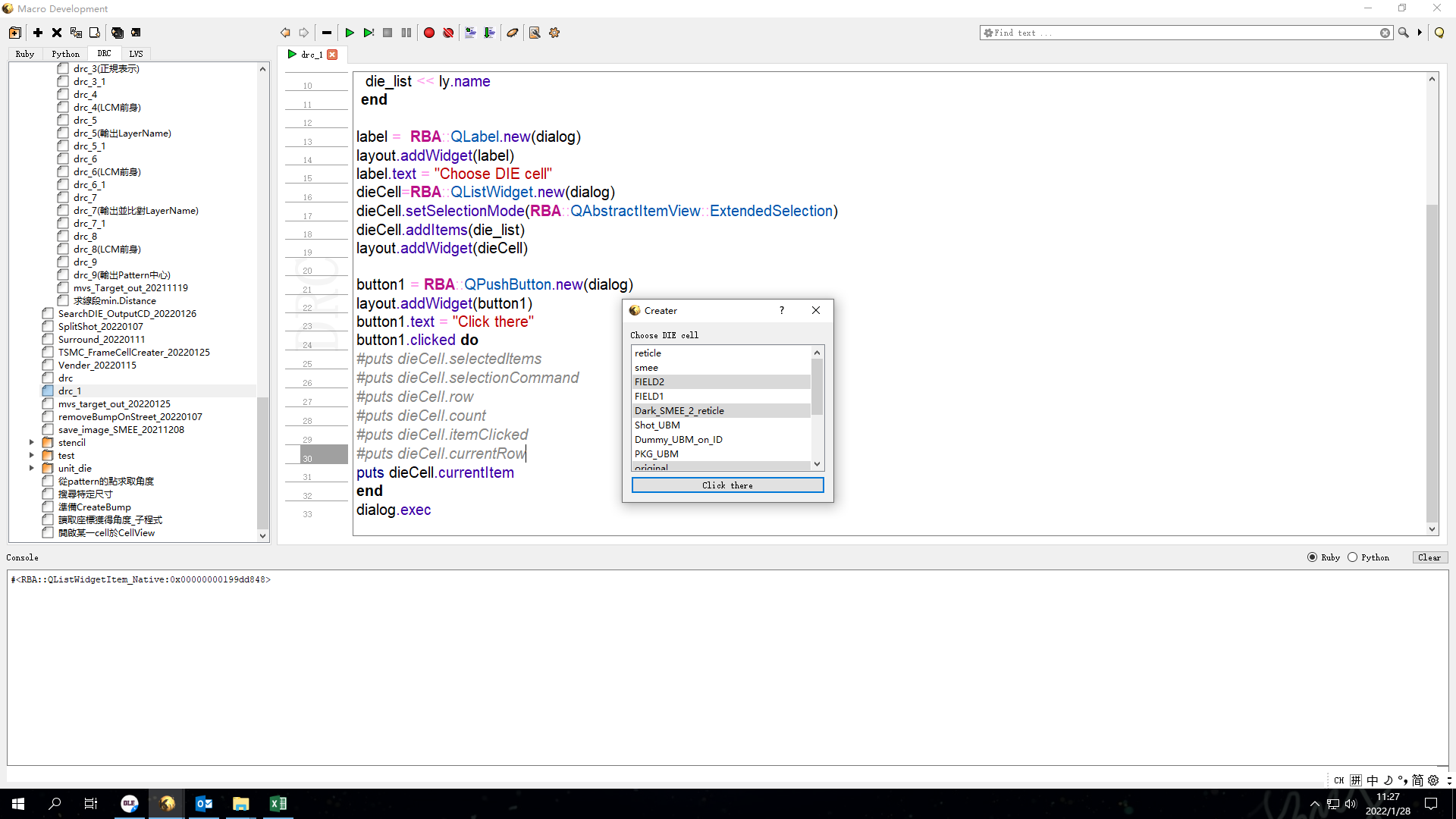• "selectedItems" is the right way ... but you need to collect the "text" attributes.

Here is an example:

``````class MultiSelectionDialog < RBA::QDialog

def initialize(parent)

super(parent)

layout = RBA::QHBoxLayout::new(self)

list = RBA::QListWidget.new(self)
list.setSelectionMode(RBA::QAbstractItemView::ExtendedSelection)
list.addItems([ "one", "two", "three", "four", "five" ])

@list = list

end

def selected_strings
@list.selectedItems.collect { |item| item.text }
end

end

dialog = MultiSelectionDialog::new(nil)
dialog.exec

puts("Selected strings: " + dialog.selected_strings.join(","))
``````

Matthias

• Hi Matthias,
Got it , Thanks.